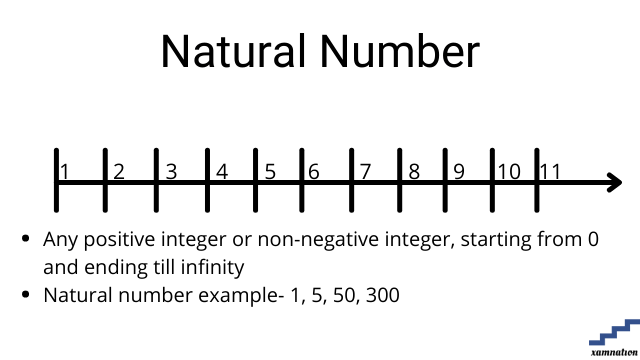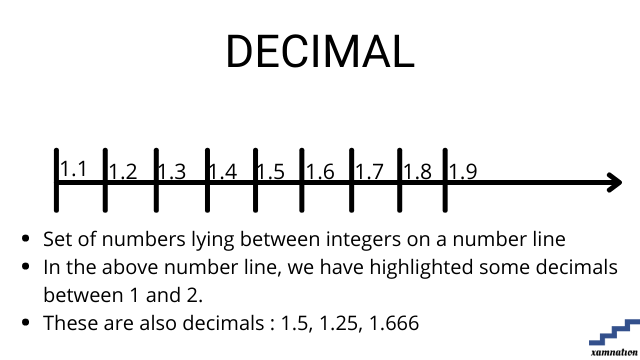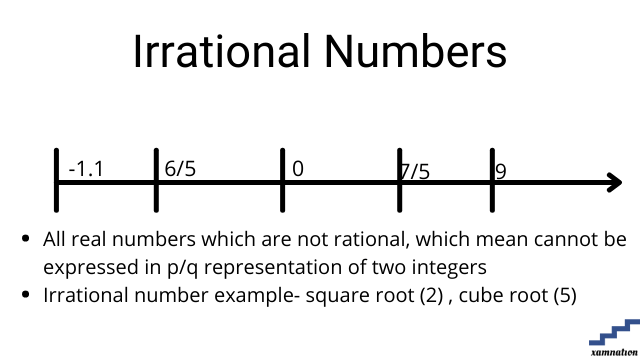# Algebra in Real Life | Practical examples of Algebra

Math is fun, when we can relate mathematics to the real world.  As we know student learn with the help of stories easily, we have created a small story case to make student understand basic algebra, and visualize its usage. This can be used as reference for school algebra syllabus.

## Real life examples of Algebra

Algebra is mainly divided into using different types of numbers, creating algebraic expressions and using mathematical operations for solving for unknown variable. Algebra has great usage in almost other mathematical topics like geometry, mensuration, trigonometry, calculus etc.

Note: Check Algebra lessons for reference to the topics mentioned in this article.

### Real life examples- Types of numbers

Scenario – Weekly supermarket visit

Remember your last visit to supermarket (or grocery store). You need to make purchase of certain items, and came there with your shopping list. These numbers of items are marked with numbers from 1 to 20. As we can see, these numbers of items in your list are countable and positive, so we can classify them as an example of natural numbers.As soon as you entered the store, you found there are no packets of cheese left. Someone has bought 100 packets of the cheese just before you, and you have 0 items left.  So, we can see, there is an example of whole numbers.

Now, entering into fruit sections, you went to purchase apples. Apples are being sold for Rs 100 per kilo. You picked three of them, and went to a weighing machine.  It shows reading of 1250 grams. Thus you need to pay for 1.25 kilo. This is an example of decimal numbersYou went into watermelon section. There were big melons and there were smaller melons too. It look like each big melon is twice the size of small melon. In other words, each small melon is ½ of big melon. You decided to pick one small melon then. This is an example of fraction.Once you are done with your purchase, you went to billing counter. The lady, she made bill of each item which come to Rs 2200. When you checked your pocket, you saw only Rs 2000 note. You were short of Rs 200, or you have negative balance of Rs 200. Then you decided to remove mangoes (which were costing you Rs 300) from your shopping cart. This is an example of both positive integer and negative integer.When coming out of super market, you thought of going diagonally, instead of going straight and then taking right turn to your car. Your teacher had just taught you properties of triangle, you can observe that your diagonal will be shorter than going straight and then taking right. You can also calculate diagonal length using Pythagoras theorem, which define diagonal as square root of sum of square of two side of a right angled triangle. Thus in this calculation, you will find example of irrational number in the form of square root.We have already seen example of rational number as in example of fraction.

### Real life examples – Using mathematical operators

In the above examples, when you made a purchase and billing was being done, you will observe the use of mathematic operations.  For example- Billing counter lady will find the price of apples by multiplying 1.25 with 100. She will sum all values to find the total bill amount. And when you gave her Rs 2000, she will return back the balance money.  We also see example of BODMAS theorem, because in billing, we will first multiply to find price of individual item, and then add them to find total bill, and then subtract from Rs 2000 to find the balance amount.

### Real life examples –finding unknown value

Now, when going from super market to your home, you find that there is very little petrol left in your car. Your car drive for 20 km in one litre of petrol, and there is hardly 2 litres of petrol left. Your home is 80 kilometer from super market.

Equation that you will create for solving this algebraic expression will be.

Y = 80/20 – 2     (where y is petrol needed)

You create a mathematical equation to find out how much petrol you need for refill to reach home. As your home is 80 km, so your car will need 4liter of petrol. Since you had only 2 liters left, you need to go to petrol station, to add 2 liters more in your car to reach home.

As, you can see, you can solve these unknown variable of petrol , and thus reached home from supermarket. This is an example of creating mathematical equation and finding unknown values.

## End note

In this article, we have tried to present the mathematics in story examples format, and giving practical examples for topics of algebra. We earlier found that lots of students fear math, as they find difficult to understand different terms and operations in algebra, and also in other topics of mathematics.

We hope you must have understood the examples of algebra in real life in this article. You can share your feedback, comment or your doubt with us. We will glad to assist you. We will be coming with real life story of higher order algebra in another article.